# In the lab, you measure the initial rate of an enzyme reactionas a function of...

In the lab, you measure the initial rate of an enzyme reaction as a function of substrate concentration in the presence and absence of an unknown inhibitor (0.05 mM). The following data are obtained when the total enzyme concentration is 1 x 10-6mM:

 [S] mM 0.0001 0.0002 0.0005 0.001 0.002 0.005 0.01 0.02 0.05 0.1 0.2 NoInhibitor V0 (mM/min) 33 50 71 83 91 96 98 99 100 100 100 WithInhibitor V0 (mM/min) 17 29 50 67 80 91 95 98 99 100 100

A) What are Vmax and Km in the absence of inhibitor? (2 marks)

B) What kind of inhibitor is it likely to be and why? (1 mark)

C) Calculate the inhibition constant for this inhibitor with the enzyme. (3 marks)

D) Calculate the turnover number and specificity constant for this enzyme. (2 marks)

E) When [S] = 0.0004, what will V0 be in the i) absence ii) presence of inhibitor? (2 marks)

Step 1: Calculate the reciprocals of [S] and V0 to obtain 1/[S] and 1/V0

 Given Data [S] mM V0 without inhibitor V0 with inhibitor 0.0001 33 17 0.0002 50 29 0.0005 71 50 0.001 83 67 0.002 91 80 0.005 96 91 0.01 98 95 0.02 99 98 0.05 100 99 0.1 100 100 0.2 100 100 Calculate the reciprocals of [S] and V0 1/[S] 1/V without inhibitor 1/V with inhibitor 10000 0.030 0.059 5000 0.020 0.034 2000 0.014 0.020 1000 0.012 0.015 500 0.011 0.013 200 0.010 0.011 100 0.010 0.011 50 0.010 0.010 20 0.010 0.010 10 0.010 0.010 5 0.010 0.010

Step 2: Plot a graph between 1/[S] and 1/V0 to obtain the Lineweaver-Burk plot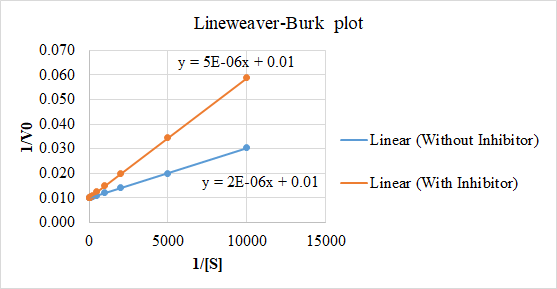Step 3: Obtain the regression equations for both the plots (see image) which appears in the form of y = mx + c (or) y = (km/Vmax).1/[S] + 1/Vmax. The reciprocal of y-intercept gives Vmax. Multiplying this Vmax with slope m gives Km

 Parameter Without inhibitor With inhibitor Regression equation y = 2E-06x + 0.01 y = 5E-06x + 0.01 1/Vmax 0.01 0.01 Vmax 100 100 Km/Vmax 0.00 5.00E-06 Km 2.00E-04 5.00E-04 This is Km This is Km apparent

Based on the above calculations, find the answers below for the given questions

A) What are Vmax and Km in the absence of inhibitor?

Vmax = 100 mM/min and Km = 2.00E-04 (or) 2 x 10-4 mM

B) What kind of inhibitor is it likely to be and why?

This is a competitive inhibition because the plots are converging on y-axis; Km value increased with increase in inhibitor concentration; Vmax is constant irrespective of the inhibitor concentration

C) Calculate the inhibition constant for this inhibitor with the enzyme.

The inhibition constant Ki is calculated using the formula

Ki = Km . [I] / (Km apparent - Km) (Here Km apparent can be found in Step 3)

= (2 x 10-4) . 0.05 / ((5 x 10-4) - (2 x 10-4)) = 13.33 mM

D) Calculate the turnover number and specificity constant for this enzyme.

The turnover number (Kcat) is calculated as

Kcat = Vmax /Et (where Et is the total enzyme concentration)

= 100/10-6 = 108 / min

Specificity constant is given as Kcat/Km = 108/(2 x 10-4) = 5 x 1011 min/mM

E) When [S] = 0.0004, what will V0 be in the i) absence ii) presence of inhibitor?

[S] = 0.0004 or 1/[S] = 2500. Calculate 1/V0 using the above regression equations, i.e.,

In the absence of inhibitor y = 2E-06x + 0.01 (Here y = 1/V0 and x= 1/[S])

So 1/V0 = [(2 x 10-6). 2500] + 0.01 = 0.015

V0 = 1/0.015 = 66.67 mM/min

Similarly,

In the presence of inhibitor y = 5E-06x + 0.01

So 1/V0 = [(5 x 10-6). 2500] + 0.01 = 0.0225

V0 = 1/0.0225 = 44.44 mM/min

#### Earn Coin

Coins can be redeemed for fabulous gifts.

Similar Homework Help Questions
• ### how did they get these answers? we Vulins Hemoglobin/Am 1. An allosteric interaction between a) binding of a molecul...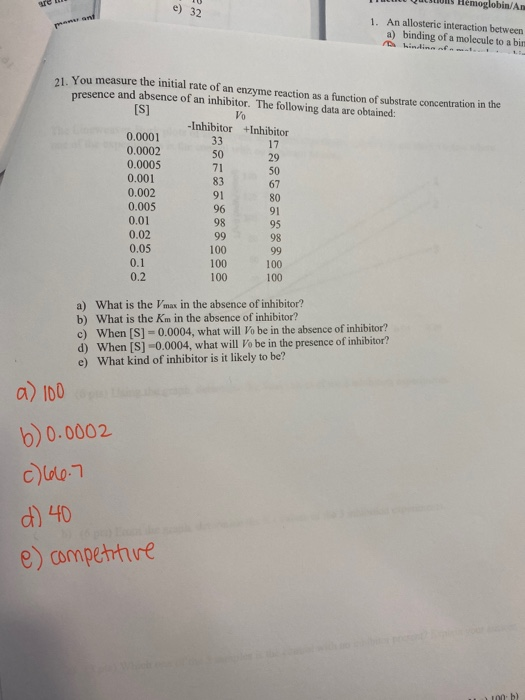how did they get these answers? we Vulins Hemoglobin/Am 1. An allosteric interaction between a) binding of a molecule to a bir 0 You measure the initial rate of an enzyme reaction as a function of substrate concentration in the presence and absence of an inhibitor. The following data are obtained: [S] Vo -Inhibitor +Inhibitor 17 29 0.0001 0.0002 0.0005 0.001 0.002 0.005 0.01 0.02 0.05 0.1 100 100 100 98 99 100 100 0.2 a) What is the Vmax...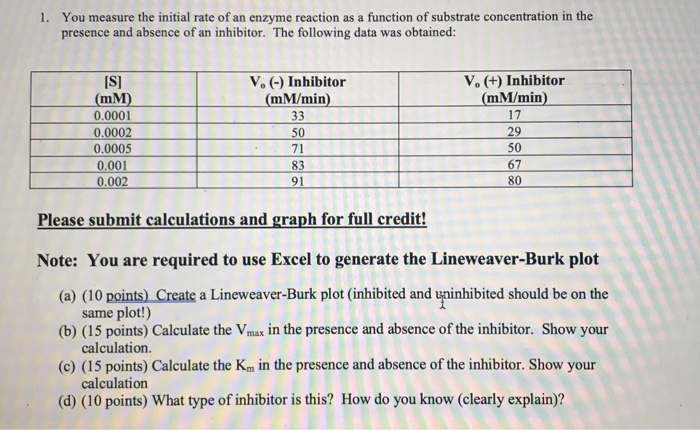need B C and D done please please please help!!! 1. You measure the initial rate of an enzyme reaction as a function of substrate concentration in the presence and absence of an inhibitor. The following data was obtained: V. (-) Inhibitor (mm/min) (+) Inhibitor (mM/min) 17 [S] (MM) 0.0001 0.0002 0.0005 0.001 0.002 Please submit calculations and graph for full credit! Note: You are required to use Excel to generate the Lineweaver-Burk plot (a) (10 points) Create a Lineweaver-Burk...

• ### The kinetics of an enzyme is measured as a function of substrate concentration in the presence...

The kinetics of an enzyme is measured as a function of substrate concentration in the presence and absence of 2 mM inhibitor. The data from the experiments are shown below: [S] (uM) Vo ( uM/min ) Vo ( uM/min ) with 2 mM inhibitor. [S]   (mM)                     Vo ( mM/min )            Vo ( mM/min ) with 2 mM inhibitor 3                                    10.4                            4.1 5                                    14.5                            6.4 10                                  22.5                           11.3 30                                  33.8                           22.6 90                                  40.5                           33.8 a). Please draw a set of Vo...

• ### You measure the initial rate of an enzyme reaction as a function of substrate concentration in...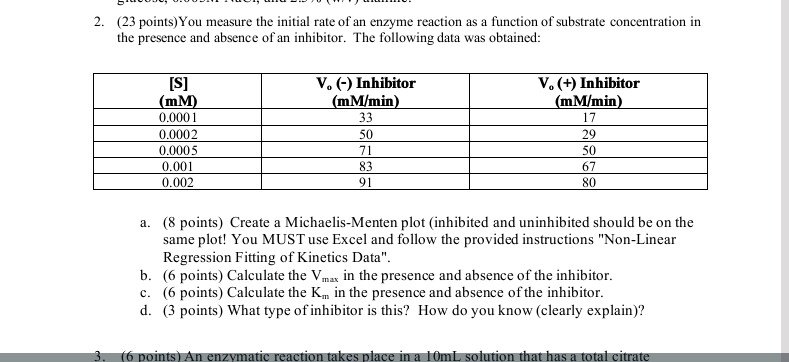You measure the initial rate of an enzyme reaction as a function of substrate concentration in the presence and absence of an inhibitor. The following data was obtained: Create a Michaelis-Menten plot (inhibited and uninhibited should be on the same plot! You MUST use Excel and follow the provided instructions "Non-Linear Regression Fitting of Kinetics Data". Calculate the V_max in the presence and absence of the inhibitor. Calculate the K_m in the presence and absence of the inhibitor. What type...

• ### 2. The table shows the kinetic data for a reaction catalyzed by an enzyme under the...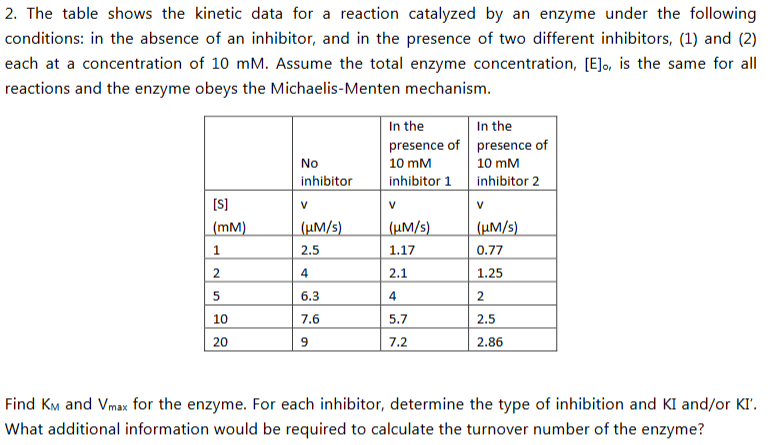2. The table shows the kinetic data for a reaction catalyzed by an enzyme under the following conditions: in the absence of an inhibitor, and in the presence of two different inhibitors, (1) and (2) each at a concentration of 10 mM. Assume the total enzyme concentration, [Elo, is the same for all reactions and the enzyme obeys the Michaelis-Menten mechanism In the presence of presence of 10 mM inhibitor 1 inhibitor2 In the 10 mM No inhibitor mM 2.5...

• ### The following data was obtained for an enzyme in the absence of an inhibitor, and in...

The following data was obtained for an enzyme in the absence of an inhibitor, and in the presence of two different inhibitors. The concentration of each inhibitor was 10 mM. The total concentration of enzyme was the same for each experiment. [S] {mM} without inhibitor v, {umol/(ml*s)} with inhibitor A v, {umol/(ml*s)} With inhibitor B v, {umol/(ml*s)} 0.0 0.0 0.0 0.0 1.0 3.6 3.2 2.6 2.0 6.3 5.3 4.5 4.0 10.0 7.8 7.1 8.0 14.3 10.1 10.2 12.0 16.7 11.3...

• ### The table below lists initial velocities measured for an enzymatic reaction at different substrate concentrations in...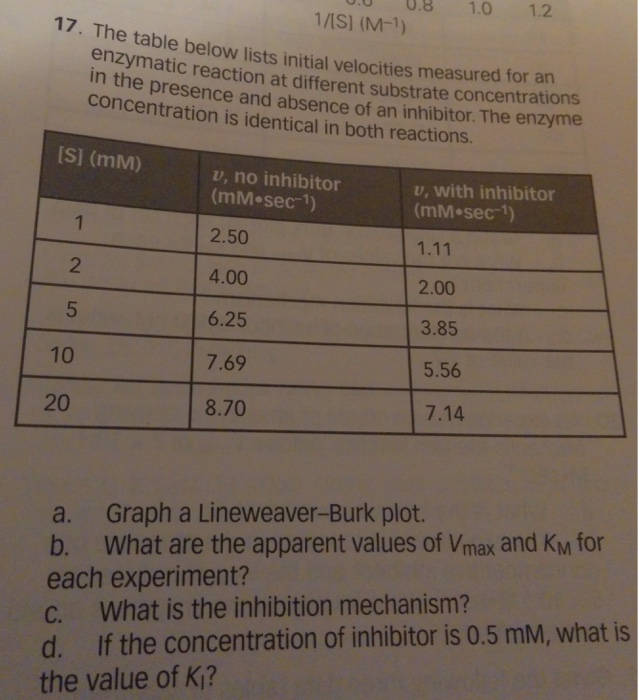The table below lists initial velocities measured for an enzymatic reaction at different substrate concentrations in the presence and absence of an inhibitor. The enzyme concentration is identical in both reactions. Graph a Lineweaver-Burk plot. What are the apparent values of vmax and km for each experiment? what is the inhibition mechanism If the concentration of inhibitor is 0.5 mM, what is the value of K1?

• ### Study on Dehydrogenase

Case 13: Inhibition of AlcoholDehydrogenase Focus concept: The inhibition of the alcohol dehydrogenase by a formamide compound is examinedQuestions3. The authors of this study studied the ability of N-1,5-dimethylhexylformamide to inhibit mouseADH1. The activity of the enzyme was measured in the absence of inhibitor, and in the presence of 1.0 :M inhibitor. The data are presented in Table 13.1.a. What are the KM and Vmax values for ADH in the absence of inhibitor? in the presence of the inhibitor?Table 13.1:...

• ### Biochemistry. Please answer all completely. 13. Identify the electrophilic center and nucleophile in the below image...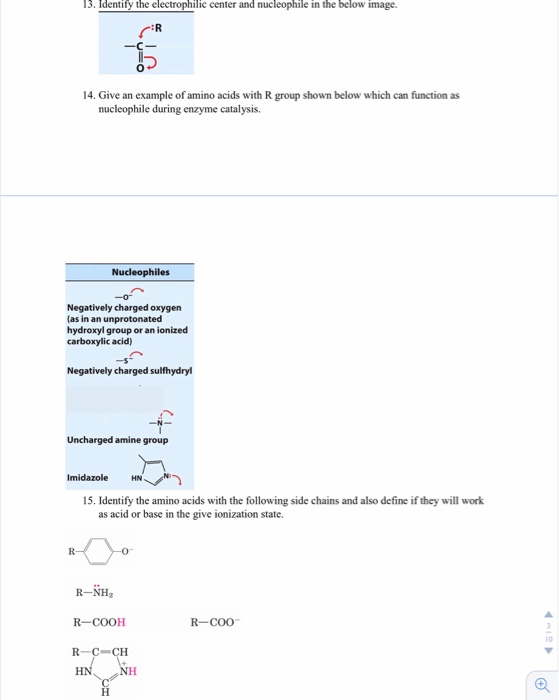Biochemistry. Please answer all completely. 13. Identify the electrophilic center and nucleophile in the below image : R 14. Give an example of amino acids with R group shown below which can function as nucleophile during enzyme catalysis. Nucleophiles Negatively charged oxygen (as in an unprotonated hydroxyl group or an ionized carboxylic acid) Negatively charged sulfhydryl Uncharged amine group HN Imidazole 15. Identify the amino acids with the following side chains and also define if they will work as acid...

• ### 5) (14 marks) The following kinetic data were obtained for an enzyme in the absence of...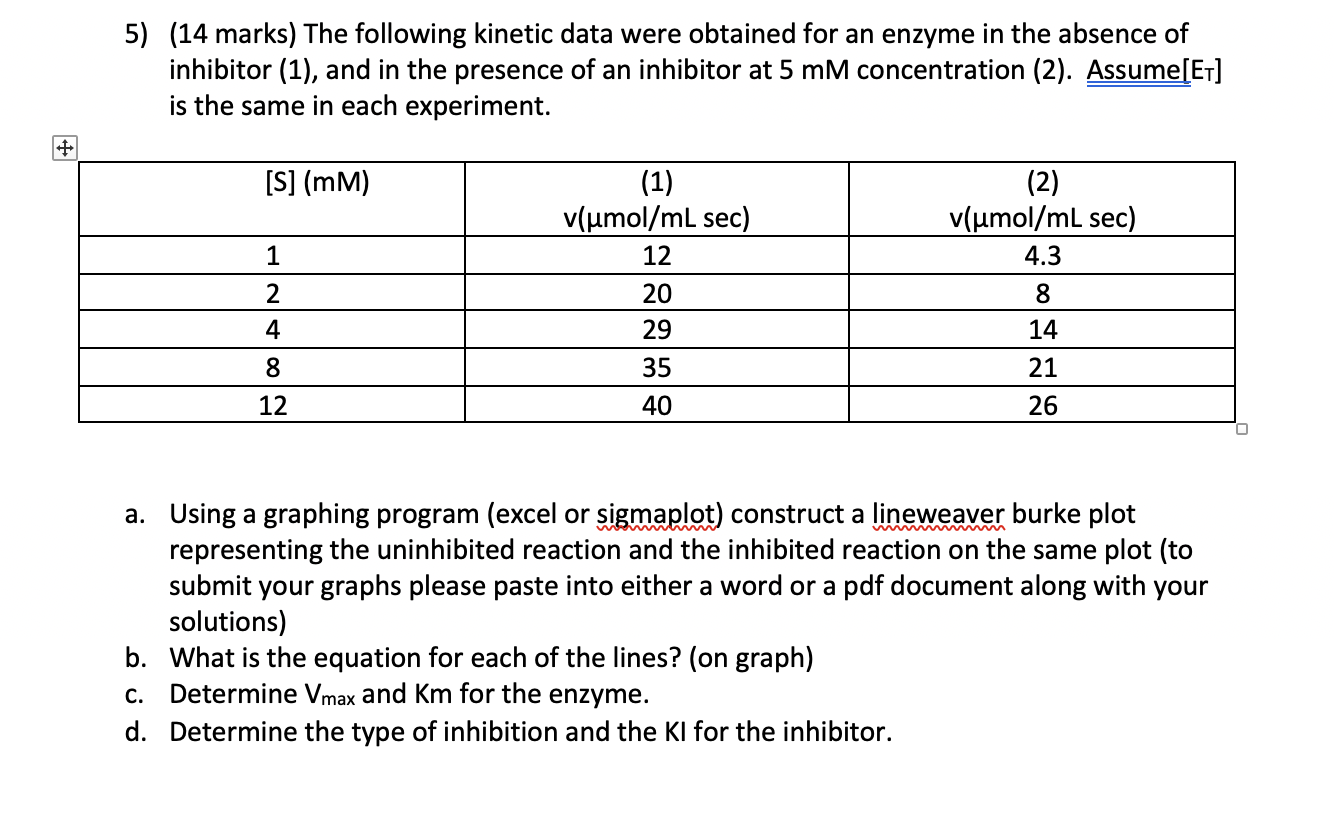5) (14 marks) The following kinetic data were obtained for an enzyme in the absence of inhibitor (1), and in the presence of an inhibitor at 5 mM concentration (2). Assume[ET] is the same in each experiment. [S] (MM) (1) v(umol/mL sec) 12 (2) v(umol/mL sec) 4.3 1 8 2 4 20 29 14 21 8 35 12 40 26 a. Using a graphing program (excel or sigmaplot) construct a lineweaver burke plot representing the uninhibited reaction and the inhibited...